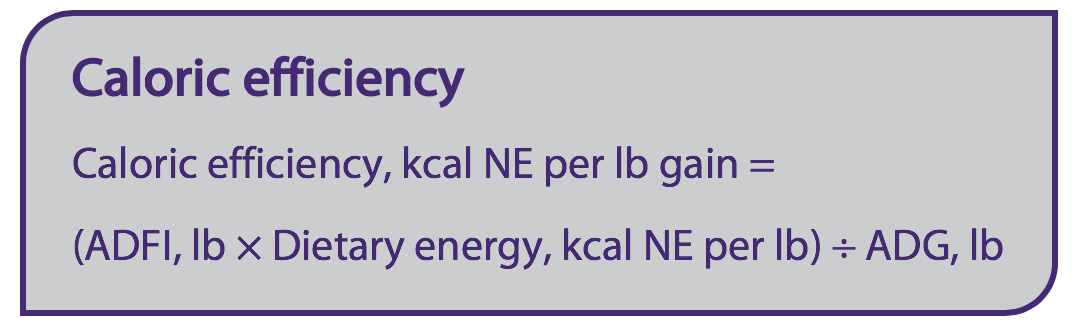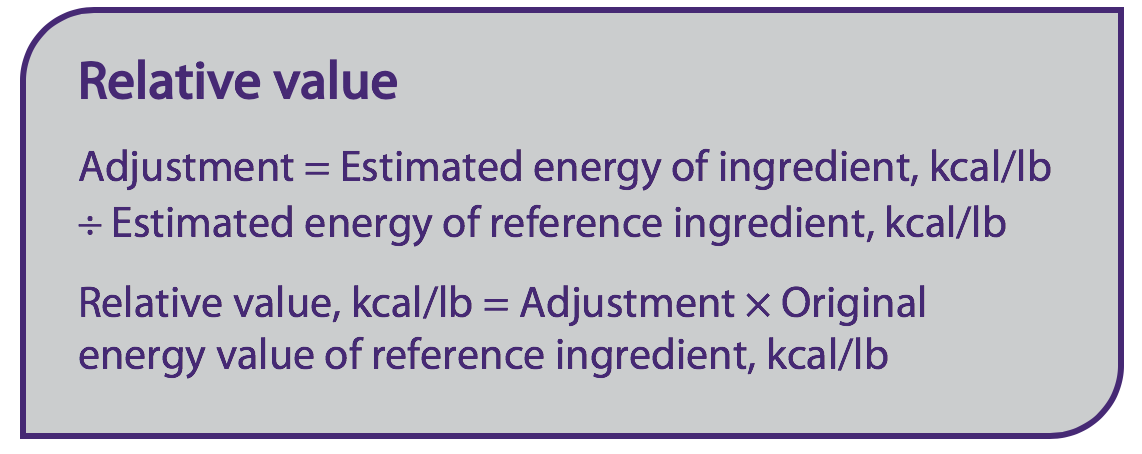# Kansas State University

search
1. K-State home
2. »College of Agriculture
3. »ASI
4. »Research and Extension
5. »Swine
6. »SwineNutritionGuide
7. »Assigning energy values

## Animal Sciences and Industry

### Assigning energy values

Dietary energy is the most expensive component of swine diets. Precision in assigning the energy value of a feed ingredient is crucial to achieve the predicted performance and optimal feed cost.

Energy can be expressed as digestible (DE), metabolizable (ME), or net energy (NE). The DE and ME energy systems are the most widely used for evaluating ingredients because energy values are relatively easy to measure. However, the NE system is recognized as the closest ingredient energy value estimate because it takes the heat increment from digestive process and metabolism of feeds into account. Importantly, the same energy system must be consistently used across the feed ingredients used in diet formulation.

Practical approaches can be used to assign energy values to feed ingredients (Gonçalves et al., 2016).

#### Prediction equations

Prediction equations generally require chemical analysis input for dry matter (DM), crude protein (CP), ether extract (EE), neutral detergent fiber (NDF) or acid detergent fiber (ADF), ash, and starch to yield a predicted energy value. For an accurate ingredient analysis, following a standardized sampling procedure is key.

The NRC and the Brazilian Tables present equations for predicting NE developed by Noblet (Noblet et al., 1994). EvaPig® is a software developed by INRA in partnership with the French Association for Animal Production (AFZ) and Ajinomoto Eurolysine S.A.S. that presents equations for predicting NE based on databases from these organizations. The prediction equations for NE from INRA and the Brazilian Tables account for difference in energy digestibility between growing pigs and sows, while the NRC equations do not.

#### Validation experiments

Validation experiments are conducted to confirm energy values for feed ingredients (Li et al., 2017). In these experiments, the ingredient is included in increasing amounts in the diet while other nutrients are maintained at a constant level among dietary treatments. This is based on the fact that pigs tend to consume feed to meet the energy requirements, thus if the other nutrients such as lysine are maintained constant, similar growth performance is expected (Li et al., 2018).

Initially, an estimated energy value is assigned to the ingredient to formulate experimental diets, which is most often derived from nutritional tables. Then, feed efficiency or caloric efficiency are used to determine whether the energy value of the ingredient was accurately estimated. Caloric efficiency is the most commonly used criteria and is determined by estimating the amount of daily energy intake per pound of gain (kcal of NE per lb of gain).If the energy value of the ingredient was accurately estimated, a similar caloric efficiency is observed among the dietary treatments with increasing amounts of the ingredient. If the caloric efficiency is not similar, the energy level for the ingredient is likely underestimated if caloric efficiency improves with increasing amounts of the ingredient, or overestimated if caloric efficiency worsens (Li et al., 2017). The NE values of corn, distillers dried grains with solubles, canola meal, wheat middlings, soybean oil, tallow, among other ingredients have been validated through this method (Hastad et al., 2005; Wu et al., 2007; Adeola et al., 2013; De Jong et al., 2014; Graham et al., 2014; Nitikanchana et al., 2015; Li et al., 2017).

#### Relative values

The use of relative values can be used if 1) the feed ingredient contains a similar composition (CP, NDF, EE, ash, starch) to the reference ingredient and 2) the reference ingredient energy value is reliable (Gonçalves et al., 2016). In this method, both the new ingredient and the reference ingredient are submitted to the same approach to be assigned an energy value, which can be any of the approaches described above. The estimated energy value for the reference ingredient is then compared to its original reliable energy value. If there is a difference between estimated and original energy values, an adjustment is applied to the estimated energy value of the ingredient of interest.

For example, the estimated energy value of the ingredient of interest is 910 kcal NE/lb and the estimated energy value of corn is 1,210 kcal NE/lb, therefore the ratio is 910 ÷ 1,210 = 0.75. The adjustment is then applied on the original energy value of the reference ingredient to generate a relative energy value for the ingredient of interest. For example, if the original reliable energy value of corn is 1190 kcal NE/lb, the relative value of the ingredient of interest is 1,190 × 0.75 = 892 kcal NE/lb.